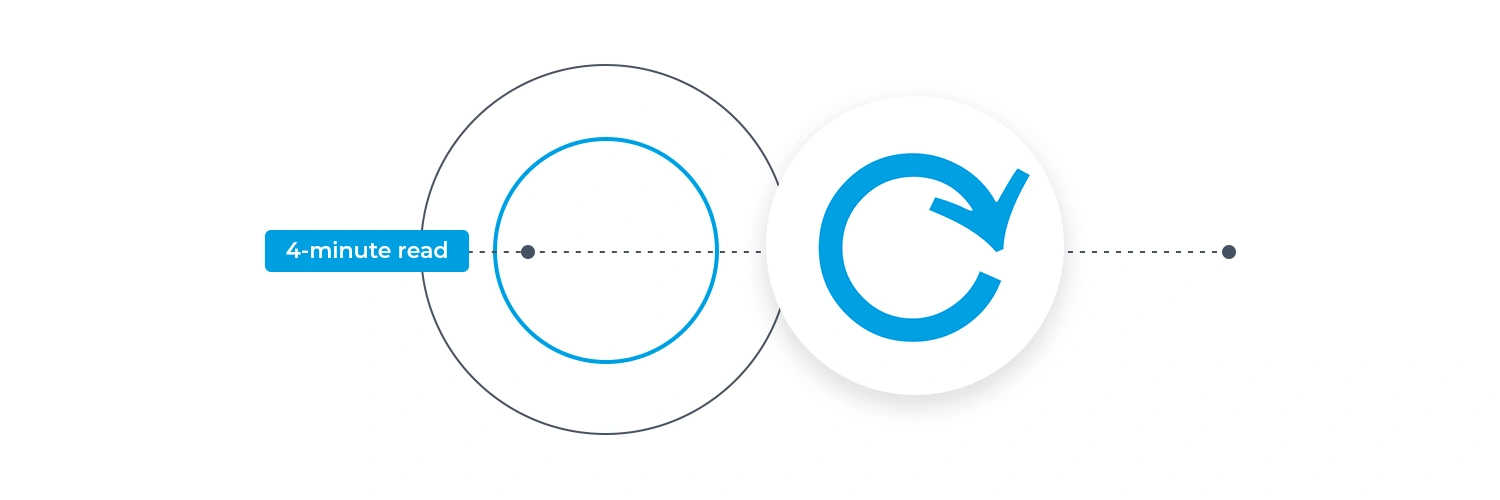# What is Iteration in Programming?

### Author: Daragh Ó Tuama

What is Iteration in Programming?In programming, the more you learn, the more you get confused. Not because of the complex topics, because no matter how complex certain topics are, you will probably get what they mean after a few practices. There are various concepts in programming, and some of their characteristics are pretty similar in function, so most tend to confuse one with another. One of such features is iteration.

Some people often confuse Iteration with recursion. Though their process may seem similar, both are used for entirely different purposes. Now, let us delve deeper into the topic of iteration programming to understand them.

## What Is Iteration?

In programming, you will define several steps to accomplish specific tasks. In some instances, you will need to continue those steps for n times until it meets a particular condition.

So what will you do:

• Enter the same code again and again till the prerequisites are met (or)
• Will you input one code and let it repeat on its own till the conditions are met

You probably will choose the second option because why would you lengthen your code by entering the same code multiple times.

This process in which the programmer enters a block of code and instructs the program to repeat the step several times till the specific condition is met is known as iteration.

## Difference Between Recursion and Iteration

Iteration is often confused with recursion. So let us give you a clear definition.

• Recursion is also repeating code, but it calls the same function again until the necessary criteria are met.
• While iteration is used for much simpler purposes, we repeat the same block of code until the conditions are met.

## Types of Loops in Programming

So how exactly is iteration implemented in a code?

Through certain features, commonly known as ‘loops’. Every programming language has two different types of loops:

• for loop
• while loop

Another type of loop is called do while loop, but it is not used in every programming language and is quite similar to the while loop.

### for Loop

The for loop is used to execute a certain block code n times till the condition is fulfilled.

The for loop has the following syntax:

for(initialization; condition; increment/decrement)

{

// loop body

}

Here,

• The initialization is where you declare any variables used to initiate the procedure. Declaring the initialization value isn’t strictly necessary. (For example, i=1).
• The condition is in the middle, and it’s here that you’ll need to specify the variable’s condition. The block of code will get executed if the specified condition is ‘true.’ If it’s a ‘false,’ the loop is skipped, and the programme proceeds to the following statement.
• The control jumps to the increment/decrement portion when the loop’s body is executed. The variable is either increased or decremented here.

Following the increment or decrement operation, the control returns to the condition to determine whether or not the new variable is true. This approach is repeated until all of the criteria have been met.

### while Loop

The while is used to execute a code block as long as the condition is true.

The while loop has the following syntax:

initialization

while (condition)

{

// loop body

// increment/decrement

}

Here,

• The initialization is declared before the loop.
• The variable is verified in the condition. If it’s a ‘true,’ the loop body is executed; if it’s a ‘false,’ control is passed to the following statement.
• The increment/decrement is where the variable value is either incremented or decremented.

The operation runs until the provided criteria are met, similar to a loop.

## Examples of Iteration in Programming

Now let’s look at some examples that can make you understand the concept more clearly:

### Example 1:

The for loop will be used in the following example to print a statement n times.

Code:

for (i = 1; i <= 5; i++) {

printf(“Iteration is an interesting concept\n”);

}

Output:

Iteration is an interesting concept

Iteration is an interesting concept

Iteration is an interesting concept

Iteration is an interesting concept

Iteration is an interesting concept

### Example 2:

In the following example, we will be employing the while loop to print the given statement n times.

Code:

int i = 1;

while (i < 6) {

printf(“Iteration is an interesting concept\n”);

i++;

}

Output:

Iteration is an interesting concept

Iteration is an interesting concept

Iteration is an interesting concept

Iteration is an interesting concept

Iteration is an interesting concept

## Wrapping Up

We hope this article helped you clear, relevant queries you had regarding iteration. If the concept of iterations is new to you, don’t let them scare you. As you get deeper into programming, these things become second nature. If you are new to programming, then try out the free 5 Day Coding Challenge, where you can learn the basics of HTML, CSS and JavaScript. It takes just one hour a day over five days. Register now through the form below.